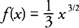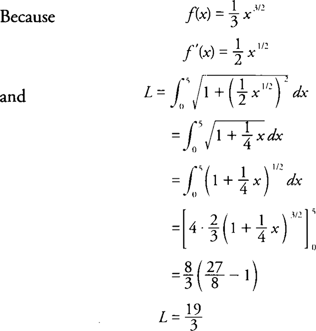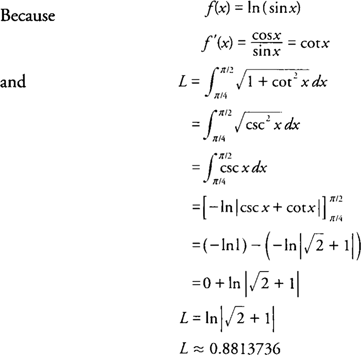## Arc Length

The length of an arc along a portion of a curve is another application of the definite integral. The function and its derivative must both be continuous on the closed interval being considered for such an arc length to be guaranteed. If y = f(x) and y′ = F'(x) are continuous on the closed interval [ a, b], then the arc length ( L) of f(x) on [ a,b] isSimilarly, if x = f(y) and x' = f'( y) are continuous on the closed interval [ a,b], then the arc length ( L) of f(y) on [ a,b] isExample 1: Find the arc length of the graph ofon the interval [0,5].Example 2: Find the arc length of the graph of f(x) = ln (sin x) on the interval [π/4, π/2].